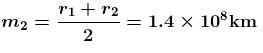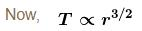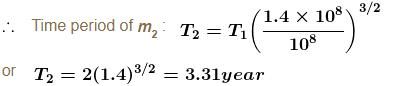Courses

# Central Forces NAT Level – 2

## 10 Questions MCQ Test Topic wise Tests for IIT JAM Physics | Central Forces NAT Level – 2

Description
This mock test of Central Forces NAT Level – 2 for Physics helps you for every Physics entrance exam. This contains 10 Multiple Choice Questions for Physics Central Forces NAT Level – 2 (mcq) to study with solutions a complete question bank. The solved questions answers in this Central Forces NAT Level – 2 quiz give you a good mix of easy questions and tough questions. Physics students definitely take this Central Forces NAT Level – 2 exercise for a better result in the exam. You can find other Central Forces NAT Level – 2 extra questions, long questions & short questions for Physics on EduRev as well by searching above.
*Answer can only contain numeric values
QUESTION: 1

### A man can jump vertically to a height of 1.5m  on the earth. Calculate the radius of a planet (in kms ) of the same mean density as that of the earth from whose gravitational field he could escape by jumping. Radius of earth is 6.41 × 106m.

Solution: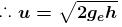For the asked planet this u should be equal to the escape velocity from its surface.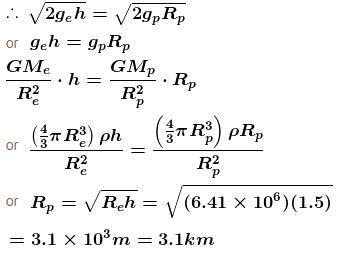*Answer can only contain numeric values
QUESTION: 2

### A particle is projected from point A, that is at a distance 4R from the centre of the earth, with speed V1 in a direction making 30° with the line joining the centre of the earth and point A, as shown in the figure. Find the speed V1. if particle passes grazing the surface of the earth. Consider gravitational interaction only between these two. (use GM/R = 6.4 × 107 m2/s2) Express your answer in the form of  (X/√2)km/sec and fill value of X.

Solution:

Conserving angular momentum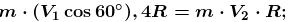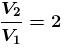Conserving energy of the system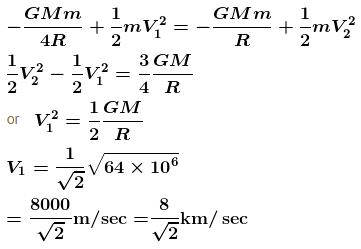*Answer can only contain numeric values
QUESTION: 3

### A cord of length 64m  is used to connect a 100kg astronaut. Estimate the value of the tension (in Newton) in the cord. Assume that the spaceship is orbiting near earth surface. Also assume that the spaceship and the astronaut fall on a straight line from the earth’s centre. The radius of the earth is 6400km.

Solution:

As according to given problem the mass of satellite M is much greater than that of astronaut m so the centre of mass of the system will be close to satellite and as the satellite is orbiting close to the surface of earth, the equation of motion of the system (S + A) will be :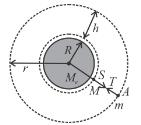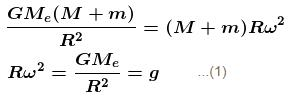And the equation motion of the astronaut will be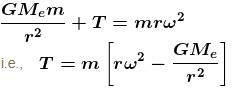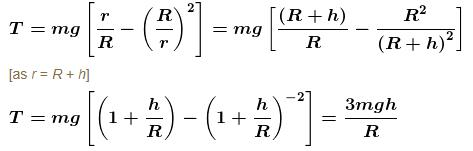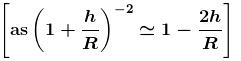So substituting the given data,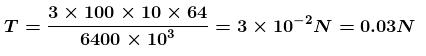*Answer can only contain numeric values
QUESTION: 4

Ravi can throw a ball a speed on earth which can cross a river of width 10m. Ravi reaches on an imaginary planet whose mean density is twice of the earth. If maximum radius of planet so that if Ravi throws the ball at same speed it may escape from planet is xkm. then x is.

(Given radius of earth = 6.4 × 106m.)

Solution:

Speed of the ball which can cross 10 m wide river is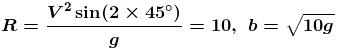Let the radius of planet is R
Then, mass of planet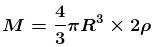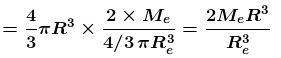Escape velocity of planet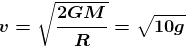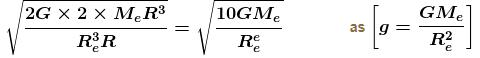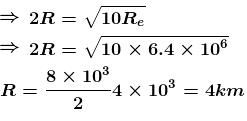*Answer can only contain numeric values
QUESTION: 5

An earth satellite is revolving in a circular orbit of radius a with velocity v0. There is a gun in the satellite and is aimed directly towards the earth. A bullet is fired from the gun with muzzle velocity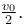Neglecting resistance offered by cosmic dust and recoil of gun, the maximum and minimum distance of bullet from the centre of earth during its subsequent motion is na and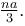Find the value of  n.

Solution:

Orbital speed of satellite is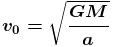From conservation of angular momentum at P and Q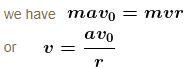From conservation of mechanical energy at P and Q, we have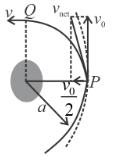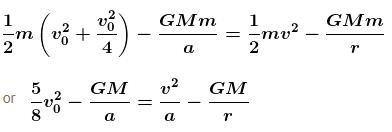Substituting values of v and v0 from Eqs. (i) and (ii), we get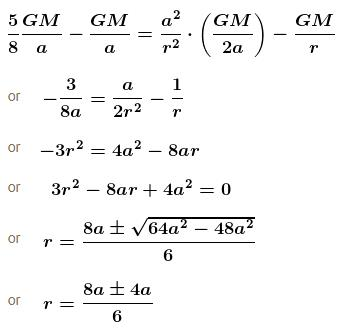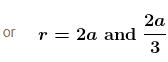Hence, the maximum and minimum distance are 2a  and  2a/3  respectively.

*Answer can only contain numeric values
QUESTION: 6

A body is projected vertically upwards from the surface of earth with a velocity sufficient to carry it to infinity. The time taken by it to reach height h is given by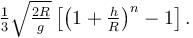Find the value of n.

Solution:

If at a distance r from the centre of the earth the body has velocity v, by conservation of mechanical energy,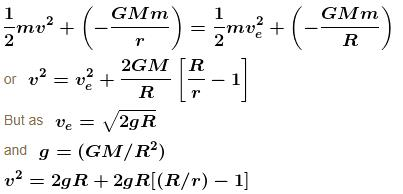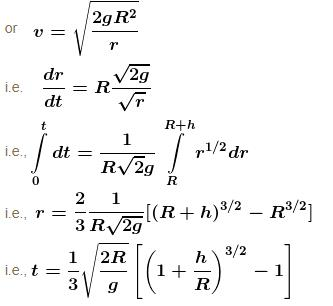*Answer can only contain numeric values
QUESTION: 7

A projectile of mass m is fired from the surface of the earth at an angle α = 60° from the vertical. The initial speed v0 is equal to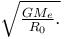The height projectile rises is given by nRe. Find the value of  n. Neglect air resistance and the earth’s rotation.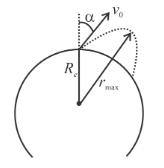Solution:

Let v be the speed of the projectile at highest point and rmax its distance from the centre of the earth. Applying conservation of angular momentum and mechanical energy,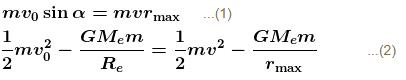Solving these two equation with the given data we get,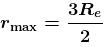or the maximum height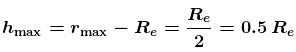*Answer can only contain numeric values
QUESTION: 8

If a planet was suddenly stopped in its orbit supposed to be circular, it will fall onto the sun in a time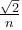times the period of the planet’s revolution. Find the value of n.

Solution:

Consider and imaginary planet moving along a strongly extended flat ellipse, the extreme points of which are located on the planet’s orbit and at the centre of the sun. The semi-major axis of the orbit of such a planet would apparently be half the semi-major axis of the planet’s orbit. So the time period of the imaginary planet T' according to Kepler’s law will be given by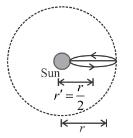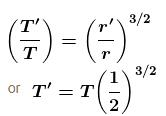∴ Time taken by the planet to fall onto the sun is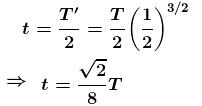*Answer can only contain numeric values
QUESTION: 9

An artificial satellite is moving in a circular orbit around the earth with a speed equal to half the magnitude of escape velocity from the surface of earth. (Radius of the earth = 6400km ). If the satellite is stopped suddenly in its orbit and allowed to fall freely on the earth, find the speed (in  m/s) with which it hits the surface of earth.

Solution: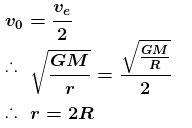or h - r - R = R or height = radius of earth.
Increase in kinetic energy = decrease in potential energy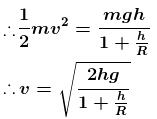Substituting the values we have,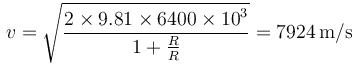*Answer can only contain numeric values
QUESTION: 10

Two planets of equal mass orbit a much more massive star (figure). Planet mmoves in a circular orbit of radius 1 × 108km with period 2year. Planet m2 moves in an elliptical orbit with closed distance r1 = 1 × 108km and farthest distance r2 = 1 × 108km, as shown.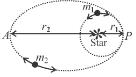Solution:

Mean radius of planet,